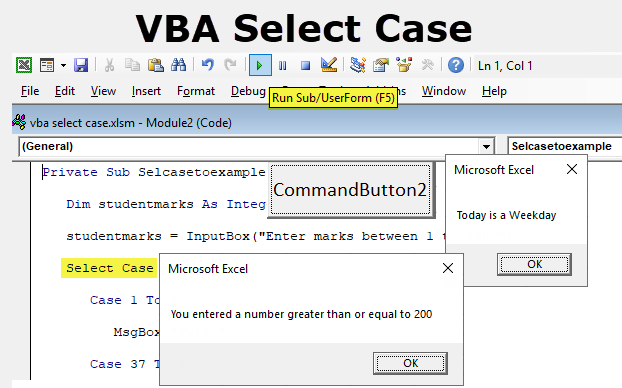# How To Use Excel VBA Select Case

Oct 17, 2020 • edited Oct 20, 2020Instead of multiple If Then statements in Excel VBA, you can use the Select Case structure.

Situation:Place a command button on your worksheet and add the following code lines:

1. First, declare two variables. One variable of type Integer named score and one variable of type String named result.

Dim score As Integer, result As String

2. We initialize the variable score with the value of cell A1.

score = Range("A1").Value

3. Add the Select Case structure.

Select Case score

Case Is >= 80

result = "very good"

Case Is >= 70

result = "good"

Case Is >= 60

result = "sufficient"

Case Else

result = "insufficient"

End Select

Explanation: Excel VBA uses the value of the variable score to test each subsequent Case statement to see if the code under the Case statement should be executed.

4. Write the value of the variable result to cell B1.

Range("B1").Value = result

5. Test the program.

Result when you click the command button on the sheet:Note: Excel VBA executes the code under the second Case statement for all values greater than or equal to 70 and less than 80.

#Tutorial#How To#VBA#If Then Statement

How To Split Text String At Specific Character In Excel

How To Use Resize Property In Excel VBA